# Resources tagged with: Odd and even numbers

Filter by: Content type:
Age range:
Challenge level:

### There are 61 results

Broad Topics > Properties of Numbers > Odd and even numbers### Next-door Numbers

##### Age 5 to 7Challenge Level

Florence, Ethan and Alma have each added together two 'next-door' numbers. What is the same about their answers?### Square Subtraction

##### Age 7 to 11Challenge Level

Look at what happens when you take a number, square it and subtract your answer. What kind of number do you get? Can you prove it?### Odd Times Even

##### Age 5 to 7Challenge Level

This problem looks at how one example of your choice can show something about the general structure of multiplication.### Sorting Numbers

##### Age 5 to 7Challenge Level

Can you sort numbers into sets? Can you give each set a name?### Always, Sometimes or Never? Number

##### Age 7 to 11Challenge Level

Are these statements always true, sometimes true or never true?### Take Three Numbers

##### Age 7 to 11Challenge Level

What happens when you add three numbers together? Will your answer be odd or even? How do you know?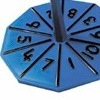### Our Numbers

##### Age 5 to 7Challenge Level

These spinners will give you the tens and unit digits of a number. Can you choose sets of numbers to collect so that you spin six numbers belonging to your sets in as few spins as possible?### The Set of Numbers

##### Age 5 to 7Challenge Level

Can you place the numbers from 1 to 10 in the grid?### The Thousands Game

##### Age 7 to 11Challenge Level

Each child in Class 3 took four numbers out of the bag. Who had made the highest even number?### Multiplication Series: Number Arrays

##### Age 5 to 11

This article for teachers describes how number arrays can be a useful representation for many number concepts.### What Do You Need?

##### Age 7 to 11Challenge Level

Four of these clues are needed to find the chosen number on this grid and four are true but do nothing to help in finding the number. Can you sort out the clues and find the number?### Odds and Threes

##### Age 7 to 11Challenge Level

A game for 2 people using a pack of cards Turn over 2 cards and try to make an odd number or a multiple of 3.### What Number?

##### Age 5 to 7 ShortChallenge Level

I am less than 25. My ones digit is twice my tens digit. My digits add up to an even number.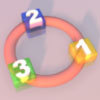### More Numbers in the Ring

##### Age 5 to 7Challenge Level

If there are 3 squares in the ring, can you place three different numbers in them so that their differences are odd? Try with different numbers of squares around the ring. What do you notice?### Magic Vs

##### Age 7 to 11Challenge Level

Can you put the numbers 1-5 in the V shape so that both 'arms' have the same total?### I Like ...

##### Age 5 to 7Challenge Level

Mr Gilderdale is playing a game with his class. What rule might he have chosen? How would you test your idea?### How Odd

##### Age 5 to 7Challenge Level

This problem challenges you to find out how many odd numbers there are between pairs of numbers. Can you find a pair of numbers that has four odds between them?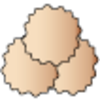### Lots of Biscuits!

##### Age 5 to 7Challenge Level

Help share out the biscuits the children have made.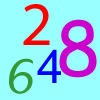### Largest Even

##### Age 5 to 7Challenge Level

How would you create the largest possible two-digit even number from the digit I've given you and one of your choice?### Down to Nothing

##### Age 7 to 11Challenge Level

A game for 2 or more people. Starting with 100, subratct a number from 1 to 9 from the total. You score for making an odd number, a number ending in 0 or a multiple of 6.### Three Spinners

##### Age 7 to 11Challenge Level

These red, yellow and blue spinners were each spun 45 times in total. Can you work out which numbers are on each spinner?### Crossings

##### Age 7 to 11Challenge Level

In this problem we are looking at sets of parallel sticks that cross each other. What is the least number of crossings you can make? And the greatest?### Number Differences

##### Age 7 to 11Challenge Level

Place the numbers from 1 to 9 in the squares below so that the difference between joined squares is odd. How many different ways can you do this?### Number Detective

##### Age 5 to 11Challenge Level

Follow the clues to find the mystery number.### Two Numbers Under the Microscope

##### Age 5 to 7Challenge Level

This investigates one particular property of number by looking closely at an example of adding two odd numbers together.### Venn Diagrams

##### Age 5 to 11Challenge Level

How will you complete these interactive Venn diagrams?### Take One Example

##### Age 5 to 11

This article introduces the idea of generic proof for younger children and illustrates how one example can offer a proof of a general result through unpacking its underlying structure.### Play to 37

##### Age 7 to 11Challenge Level

In this game for two players, the idea is to take it in turns to choose 1, 3, 5 or 7. The winner is the first to make the total 37.### Part the Piles

##### Age 7 to 11Challenge Level

Try to stop your opponent from being able to split the piles of counters into unequal numbers. Can you find a strategy?### Share Bears

##### Age 5 to 7Challenge Level

Yasmin and Zach have some bears to share. Which numbers of bears can they share so that there are none left over?### Number Tracks

##### Age 7 to 11Challenge Level

Benâ€™s class were cutting up number tracks. First they cut them into twos and added up the numbers on each piece. What patterns could they see?### Always, Sometimes or Never?

##### Age 5 to 11Challenge Level

Are these statements relating to odd and even numbers always true, sometimes true or never true?### A Mixed-up Clock

##### Age 7 to 11Challenge Level

There is a clock-face where the numbers have become all mixed up. Can you find out where all the numbers have got to from these ten statements?### Grouping Goodies

##### Age 5 to 7Challenge Level

Pat counts her sweets in different groups and both times she has some left over. How many sweets could she have had?### One of Thirty-six

##### Age 5 to 7Challenge Level

Can you find the chosen number from the grid using the clues?### Light the Lights

##### Age 5 to 7Challenge Level

Investigate which numbers make these lights come on. What is the smallest number you can find that lights up all the lights?### Neighbourly Addition

##### Age 7 to 14Challenge Level

I added together some of my neighbours' house numbers. Can you explain the patterns I noticed?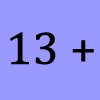### What Could it Be?

##### Age 5 to 7Challenge Level

In this calculation, the box represents a missing digit. What could the digit be? What would the solution be in each case?### Number Round Up

##### Age 5 to 7Challenge Level

Arrange the numbers 1 to 6 in each set of circles below. The sum of each side of the triangle should equal the number in its centre.### Pairs of Legs

##### Age 5 to 7Challenge Level

How many legs do each of these creatures have? How many pairs is that?### Make 37

##### Age 7 to 14Challenge Level

Four bags contain a large number of 1s, 3s, 5s and 7s. Pick any ten numbers from the bags above so that their total is 37.### Curious Number

##### Age 7 to 11Challenge Level

Can you order the digits from 1-3 to make a number which is divisible by 3 so when the last digit is removed it becomes a 2-figure number divisible by 2, and so on?### Seven Flipped

##### Age 7 to 11Challenge Level

Investigate the smallest number of moves it takes to turn these mats upside-down if you can only turn exactly three at a time.### Odd Squares

##### Age 7 to 11Challenge Level

Think of a number, square it and subtract your starting number. Is the number youâ€™re left with odd or even? How do the images help to explain this?### Sets of Numbers

##### Age 7 to 11Challenge Level

How many different sets of numbers with at least four members can you find in the numbers in this box?### Carroll Diagrams

##### Age 5 to 11Challenge Level

Use the interactivities to fill in these Carroll diagrams. How do you know where to place the numbers?### Domino Pick

##### Age 5 to 7Challenge Level

Are these domino games fair? Can you explain why or why not?### Numbers as Shapes

##### Age 5 to 7Challenge Level

Use cubes to continue making the numbers from 7 to 20. Are they sticks, rectangles or squares?### Ring a Ring of Numbers

##### Age 5 to 7Challenge Level

Choose four of the numbers from 1 to 9 to put in the squares so that the differences between joined squares are odd.### Cube Bricks and Daisy Chains

##### Age 5 to 7Challenge Level

Daisy and Akram were making number patterns. Daisy was using beads that looked like flowers and Akram was using cube bricks. First they were counting in twos.